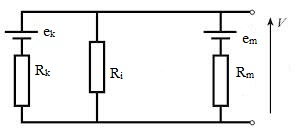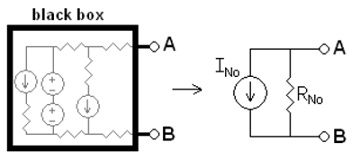#### Millman Theorem and Nortons Theorem, Physics tutorial

Millman's Theorem:

Millman's theorem or generally termed as the parallel generator theorem is a process to simplify the solution of a circuit. Particularly, Millman's theorem is employed to calculate the voltage at the ends of a circuit made up of merely branches in parallel. For highlighting, you must underscore circuit made up of just branches in parallel. This is termed after Jacob Millman, who confirmed the theorem.Assume that,

ek is the voltage generators and am be the current generators.

Ri is the resistances on the branches without generator.

Rk is the resistances on the branches having voltage generators.

Rm is the resistances on the branches having current generators.

Then Millman defines that the voltage at the ends of the circuit is provided by:

v = [Σ(±ek/Rk) + Σ(±am)}]/[Σ(1/Rk) + Σ(1/Ri)]

This can be proved by considering the circuit as a single super node. Then, according to the Ohm's and Kirchhoff's laws, the voltage between the ends of the circuit not equivalent to the net current entering the super node divided through the net equivalent conductance of the Super node? Yes it is equivalent to it.

The net current is the sum of the currents flowing in each and every branch. The total equivalent conductance of the super node is the sum of the conductance of each and every branch, as all the branches are in parallel. Whenever computing the equivalent conductance all the generators encompass to be switched off, therefore all voltage generators become short circuits and all current generators become open circuits. That is why the resistances on the branches having current generators don't appear in the expression of the net equivalent conductance.

Norton's Theorem:

Norton's theorem for the linear electrical networks that you can as well refer to as the Mayer-Norton theorem, defines that any collection of voltage sources, current source and resistors by the two terminals is electrically equivalent to an ideal current source, 'I', in parallel by a single resistor 'R'.

For a single-frequency AC system the theorem can as well be applied to the general impedances, not just resistors. The Norton equivalent is employed to symbolize any network of linear sources and impedances, at a given frequency. The circuit comprises of an ideal current source in parallel by an ideal impedance (or resistor for the non-reactive circuits).

The Norton's theorem is an expansion of Thevenin's theorem and was proposed in the year 1926 separately by two people: Hause-Siemens researcher Hans Ferdinand Mayer (1895 - 1980) and Bell Labs engineer Edward Lawry Norton (1898 - 1983).

Any black box having merely voltage sources, current sources and resistors can be transformed to a Norton equivalent circuit.

Calculation of a Norton equivalent circuit:The Norton equivalent circuit is the current source having current INo in parallel by a resistance RNo and for you to determine the equivalent you should do the given:

1) Determine the Norton current INo. Compute the output current, IAB, by a short circuit as the load (meaning 0 resistances between A and B). This is INo

2) Determine the Norton resistance RNo. If there are no dependent sources (that is, all current and voltage sources are independent), there are two processes of finding the Norton impedance RNo.

3) Compute the output voltage, VAB, if in open circuit condition (that is, no load resistor - meaning infinite load resistance). RNo equivalents this VAB divided by INo

Or you can:

4) Substitute independent voltage sources by short circuits and independent current sources by open circuits. The net resistance across the output port is the Norton impedance RNo

Or alternatively:

5) Make use of the given Thevenin's resistance: as the two are equivalent.  Though, if there are dependent sources, then you must make use of the general method.

6) Join a constant current source at the output terminals of the circuit having a value of 1 Ampere and compute the voltage at its terminals. The quotient of this voltage divided by the 1 A current is the Norton impedance RNo. This process should be employed when the circuit includes dependent sources.

Ohm's Law:

Ohm's law defines that the current via a conductor between the two points is directly proportional to the potential difference or voltage across the two points, and inversely proportional to the resistance among them.

The mathematical equation that explains the relationship is:

I = V/R

Here, 'I' is the current via the conductor in units of amperes, 'V' is the potential difference measured across the conductor in units of volts, and 'R' is the resistance of the conductor in units of ohms. More particularly, Ohm's law defines that the 'R' in this relation is constant, and independent of the current.

The law was named following the German physicist Georg Ohm, who, in a treatise introduce in the year 1827, illustrated measurements of applied voltage and current via simple electrical circuits having different lengths of wire. He represented a somewhat more complex equation than the one above to describe his experimental outcomes.

I = V/R is the modern form of the Ohm's law.

The word Ohm's law is as well employed to refer to different generalizations of the law originally formulated through Ohm and the simplest illustration of this is:

J = σE

Here, 'J' is the current density at a given position in a resistive material, 'E' is the electric field at that location, and 'σ' is a material dependent parameter termed as the conductivity. This reformulation of Ohm's law is due to the Gustav Kirchhoff.

Resistive circuits:

Resistors are circuit elements which impede the passage of electric charge in agreement by Ohm's law and are designed to encompass a specific resistance value 'R'. The element (resistor or conductor) that behaves according to the Ohm's law over some operating range is termed to as an ohmic device (or an ohmic resistor) as Ohm's law and a single value for the resistance suffice to explain the behavior of the device over that range.

Ohm's law holds for circuits having mere resistive elements and you must keep in mind that no capacitances or inductances are permitted for all forms of driving voltage or current, in spite of of whether the driving voltage or current is constant (DC) or time-varying like AC. At any time instant Ohm's law is valid for these circuits.

Resistors that are in series or in parallel might be grouped altogether into a single equivalent resistance in order to apply the Ohm's law in examining the circuit.

Reactive circuits with time-varying signals:

If reactive elements like capacitors, inductors and transmission lines are comprised in a circuit to which AC or time-varying voltage or current is applied, the relationship among voltage and current becomes the solution to a differential equation, thus Ohm's law (as stated above) doesn't directly apply since that form contains merely resistances having value 'R', not complex impedances which might have capacitance (C) or inductance (L).

Equations for time-invariant AC circuits take the similar form as Ohm's law, though, the variables are generalized into complex numbers and the voltage and current waveforms are complex exponentials.

In this approach, a current or voltage waveform takes the form Aest, here 't' is time, 's' is a complex parameter and 'A'  is a complex scalar. In any linear time-invariant system, all of the voltages and currents can be deduced by the similar 's' parameter as the input to the system, letting the time-varying complex exponential word to be canceled out and the system explained algebraically in terms of the complex scalars in the voltage and current waveforms.

The complex generalization of resistance is impedance, generally represented by Z; this can be exhibited for an inductor:

Z = sL

And for a capacitor:

Z = 1/sC

We can now write,

V = I. Z

Here 'V' and 'I' are the complex scalars in the current and voltage correspondingly and 'Z' is the complex impedance.

Reciprocity:

Reciprocity signifies to a diversity of associated theorems comprising the interchange of time-harmonic electric current densities (sources) and the resultant electromagnetic fields in Maxwell's equations for time-invariant linear media beneath certain constraints. Reciprocity is closely associated to the theory of Hermitian operators from linear algebra, applied to the electromagnetism.

Possibly the most common and general theorem is the Lorentz reciprocity (and its different special cases like Rayleigh-Carson reciprocity), named after work by Hendrik Lorentz in the year 1896 following analogous outcomes regarding sound through Lord Rayleigh and Helmholtz (Potton, 2004). Generally, it defines that the relationship between an oscillating current and the resulting electric field is unchanged when one interchanges the points where the current is positioned and where the field is measured. For the specific case of an electrical network, it is at times phrased as the statement that currents and voltages at various points in the network can be interchanged. More technically, it follows that the mutual impedance of a first circuit due to a second is the same as the mutual impedance of the second circuit due to the first.

There is as well an analogous theorem in electrostatics, termed as the Green's reciprocity, associating the interchange of electric potential and electric charge density.

The forms of reciprocity theorems are mainly employed in numerous electromagnetic applications, like examining electrical networks and antenna systems. For illustration, reciprocity means that antennas work uniformly well as receivers or transmitters and specifically that an antenna's radiation and receiving patterns are similar. Reciprocity is as well a fundamental lemma which is employed to prove other theorems regarding electromagnetic systems, like the symmetry of the impedance matrix and scattering matrix, symmetries of Green's functions for the use in boundary-element and transfer-matrix computational processes, and also Orthogonality properties of the harmonic modes in the waveguide systems (that is, as an alternative to proving such properties directly from the symmetries of the Eigen-operators).

Tutorsglobe: A way to secure high grade in your curriculum (Online Tutoring)

Expand your confidence, grow study skills and improve your grades.

Since 2009, Tutorsglobe has proactively helped millions of students to get better grades in school, college or university and score well in competitive tests with live, one-on-one online tutoring.

Using an advanced developed tutoring system providing little or no wait time, the students are connected on-demand with a tutor at www.tutorsglobe.com. Students work one-on-one, in real-time with a tutor, communicating and studying using a virtual whiteboard technology.  Scientific and mathematical notation, symbols, geometric figures, graphing and freehand drawing can be rendered quickly and easily in the advanced whiteboard.

Free to know our price and packages for online physics tutoring. Chat with us or submit request at info@tutorsglobe.com